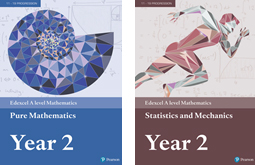Translate

An award winning sixth-form college in the heart of Central London offering a wide range of GCSE and A-Level courses

The quality of the curriculum, teaching and learners' achievements is excellent
ISI INSPECTION REPORT - APRIL 2023

T (+44) 20 7409 7273
Albemarle Independent College, 18 Dunraven Street, Mayfair, London W1K 7FE# MATHEMATICS (PEARSON)

A Level Course Outline

What is Mathematics?Mathematics is reasoning with quantities, which is why it provides ideal support to applications for so many degree courses and professions. To study A-level Mathematics you will need to have a strong command of logical thought as well as the ability to understand and manipulate numbers and quantities. Typically you may be suitable for the course if you are capable of achieving a Grade 5 or above at GCSE and you can build directly upon well learned AS-level material throughout the A-level Mathematics course.

What does the course aim to do?

Mathematics A-level will introduce you to a variety of new techniques and ideas, as well as giving you a measure of expertise in the topics which were introduced at AS-level. It builds up your logical reasoning from small steps into longer, multi-stage tasks which you must break down. This provides you with a transferrable skill and is one of the reasons that a Mathematics A-level is valued highly for degrees in the Sciences, Economics, Social Sciences, Philosophy, Engineering and, of course, Mathematics.

What topics will I study?

Most of the course is Pure Mathematics (Manipulating unknown quantities and functions, spatial reasoning etc) but one third of the course is about the application of Mathematics to the physical world of forces and motion (Mechanics), statistical data and claims (Statistics).

Pure Mathematics revisits and extends your existing understanding of functions, gradients and trigonometry as well as integrating to find areas and volumes, use of vectors in spatial reasoning and representing more complex curves with variable parameters rather than the x and y co-ordinates, amongst a few other topics.

 Mathematics (EDX) A Level SpecificationEach paper has a 2 hour exam with 100 marks and is equally weighted toward the A-level.A Level maths builds directly on AS level maths and will re-assess and extend students knowledge of several AS topics. Paper 133%and Paper 233.3% Pure Mathematics: Proof, algebra and functions, coordinate geometry in the x-y plane, sequences and series, trigonometry, exponentials and logarithms, fundamental theorem of calculus; differentiation & integration, numerical methods and vectors. Paper 333% Statistics: Statistical sampling, representing and summarising data, probability, correlation and regression, probability, statistical distributions (including normal distributions) and hypothesis testing (the null hypothesis).Mechanics: Modelling physical systems, 2D vectors, motion in a straight line, resolving forces, Newton's laws including friction, applying vectors to 2D forces.

Structure of the Course
This course will be taught for 5x 1hour lessons per week plus a weekly revision session. Homework is set at the end of each session and deadlines for submissions must be met. A full set of notes and examination questions is provided.

Enrichment
The UK Senior Mathematics Challenge is available to candidates who wish to extend and broaden their mathematical experience.

Calculator Requirement
Casio FX-991EX Scientific Calculator

 Core TextbooksEdexcel A-level Mathematics Pure MathematicsYear 2 TextbookEdexcel A-level Mathematics Statistics & Mechanics Year 2 Textbook